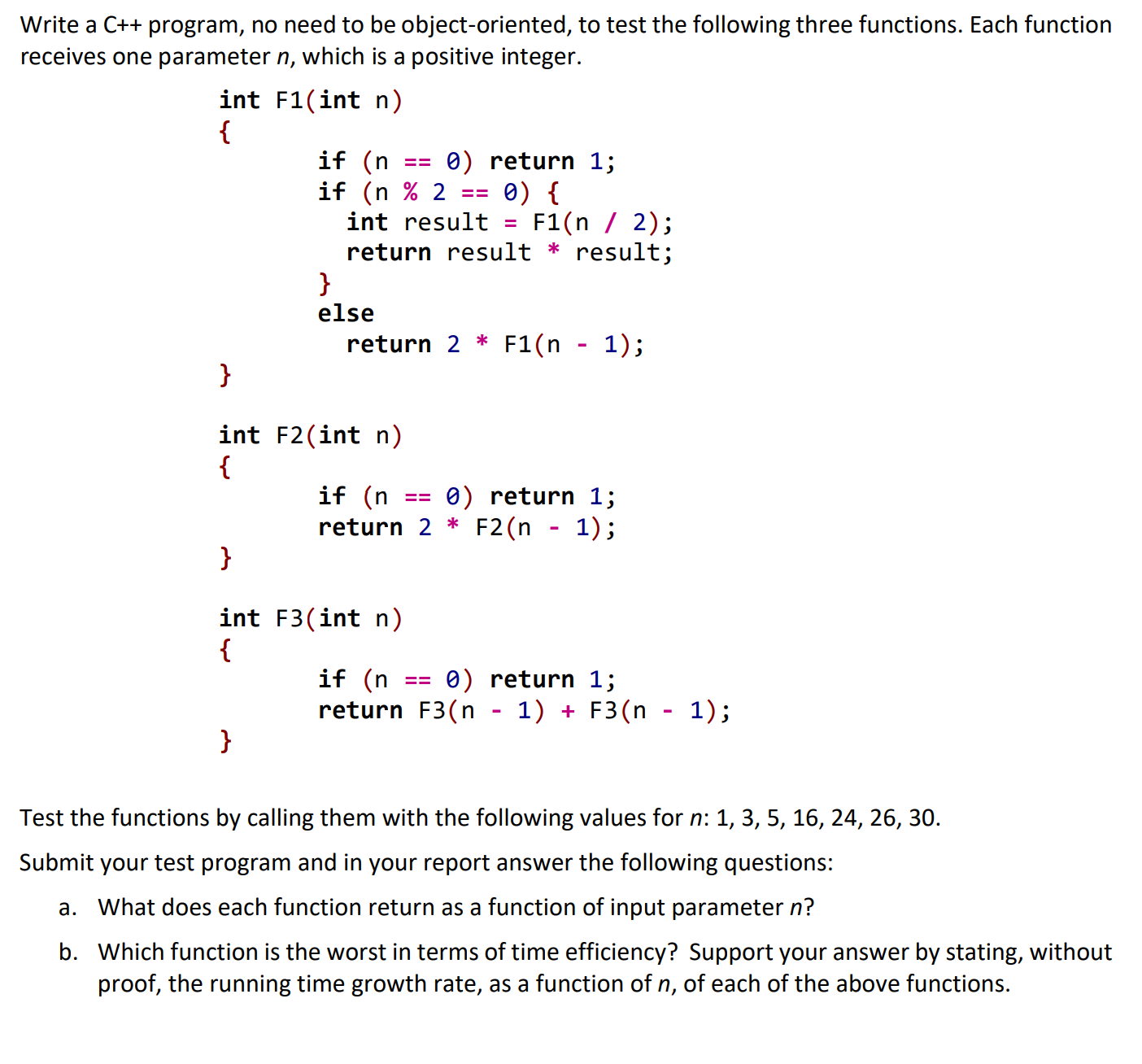# Write a C++ program, no need to be object-oriented, to test the following three functions. Each functionreceives one parameter n, which is a positive integer.int F1(int n){if (nif (n % 2int result0) return 1;0) {F1(n / 2);return result * result;else1);return 2 * F1(n}int F2(int n){if (nreturn 2 * F2(n - 1);0) return 1;int F3(int n){if (n0) return 1;return F3(n - 1) + F3(n - 1);}Test the functions by calling them with the following values for n: 1, 3, 5, 16, 24, 26, 30.Submit your test program and in your report answer the following questions:a. What does each function return as a function of input parameter n?b. Which function is the worst in terms of time efficiency? Support your answer by stating, withoutproof, the running time growth rate, as a function of n, of each of the above functions.

Question
66 viewshelp_outlineImage TranscriptioncloseWrite a C++ program, no need to be object-oriented, to test the following three functions. Each function receives one parameter n, which is a positive integer. int F1(int n) { if (n if (n % 2 int result 0) return 1; 0) { F1(n / 2); return result * result; else 1); return 2 * F1(n } int F2(int n) { if (n return 2 * F2(n - 1); 0) return 1; int F3(int n) { if (n 0) return 1; return F3(n - 1) + F3(n - 1); } Test the functions by calling them with the following values for n: 1, 3, 5, 16, 24, 26, 30. Submit your test program and in your report answer the following questions: a. What does each function return as a function of input parameter n? b. Which function is the worst in terms of time efficiency? Support your answer by stating, without proof, the running time growth rate, as a function of n, of each of the above functions. fullscreen
check_circle

Function F1

For function F1 the number of steps assuming only if branch:

T(1) = 1

T(n) = 1 + T(n/2)

Hence by repeated substitution we get

T(n) = log(n) + 1 i.e. O(logn)

For function F1 the number of steps assuming only the else branch:

T(1) = 1

T(n) = 1 + T(n-1)

Hence by repeated substitution we get

T(n) = k + T(n-k) = … = n -1 + 1 = O(n)

Hence the worst case time complexity is O(n) as O(n) is tighter than O(logn)

Function F2

For function F2 doing similar analysis as for F1 we get a worst case time complexity de...

### Want to see the full answer?

See Solution

#### Want to see this answer and more?

Solutions are written by subject experts who are available 24/7. Questions are typically answered within 1 hour.*

See Solution
*Response times may vary by subject and question.
Tagged in

### Computer Science